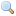# Module type Set.S

`module type S = `sig` .. `end``
Output signature of the functor `Set.Make`.

`type elt `
The type of the set elements.
`type t `
The type of sets.
`val empty : `t``
The empty set.
`val is_empty : `t -> bool``
Test whether a set is empty or not.
`val mem : `elt -> t -> bool``
`mem x s` tests whether `x` belongs to the set `s`.
`val add : `elt -> t -> t``
`add x s` returns a set containing all elements of `s`, plus `x`. If `x` was already in `s`, `s` is returned unchanged.
`val singleton : `elt -> t``
`singleton x` returns the one-element set containing only `x`.
`val remove : `elt -> t -> t``
`remove x s` returns a set containing all elements of `s`, except `x`. If `x` was not in `s`, `s` is returned unchanged.
`val union : `t -> t -> t``
Set union.
`val inter : `t -> t -> t``
Set intersection.
`val diff : `t -> t -> t``
Set difference.
`val compare : `t -> t -> int``
Total ordering between sets. Can be used as the ordering function for doing sets of sets.
`val equal : `t -> t -> bool``
`equal s1 s2` tests whether the sets `s1` and `s2` are equal, that is, contain equal elements.
`val subset : `t -> t -> bool``
`subset s1 s2` tests whether the set `s1` is a subset of the set `s2`.
`val iter : `(elt -> unit) -> t -> unit``
`iter f s` applies `f` in turn to all elements of `s`. The elements of `s` are presented to `f` in increasing order with respect to the ordering over the type of the elements.
`val fold : `(elt -> 'a -> 'a) -> t -> 'a -> 'a``
`fold f s a` computes `(f xN ... (f x2 (f x1 a))...)`, where `x1 ... xN` are the elements of `s`, in increasing order.
`val for_all : `(elt -> bool) -> t -> bool``
`for_all p s` checks if all elements of the set satisfy the predicate `p`.
`val exists : `(elt -> bool) -> t -> bool``
`exists p s` checks if at least one element of the set satisfies the predicate `p`.
`val filter : `(elt -> bool) -> t -> t``
`filter p s` returns the set of all elements in `s` that satisfy predicate `p`.
`val partition : `(elt -> bool) -> t -> t * t``
`partition p s` returns a pair of sets `(s1, s2)`, where `s1` is the set of all the elements of `s` that satisfy the predicate `p`, and `s2` is the set of all the elements of `s` that do not satisfy `p`.
`val cardinal : `t -> int``
Return the number of elements of a set.
`val elements : `t -> elt list``
Return the list of all elements of the given set. The returned list is sorted in increasing order with respect to the ordering `Ord.compare`, where `Ord` is the argument given to `Set.Make`.
`val min_elt : `t -> elt``
Return the smallest element of the given set (with respect to the `Ord.compare` ordering), or raise `Not_found` if the set is empty.
`val max_elt : `t -> elt``
Same as `Set.S.min_elt`, but returns the largest element of the given set.
`val choose : `t -> elt``
Return one element of the given set, or raise `Not_found` if the set is empty. Which element is chosen is unspecified, but equal elements will be chosen for equal sets.
`val split : `elt -> t -> t * bool * t``
`split x s` returns a triple `(l, present, r)`, where `l` is the set of elements of `s` that are strictly less than `x`; `r` is the set of elements of `s` that are strictly greater than `x`; `present` is `false` if `s` contains no element equal to `x`, or `true` if `s` contains an element equal to `x`.